# CSC 461 Lecture 17 Modeling and Virtual Track

• Slides: 24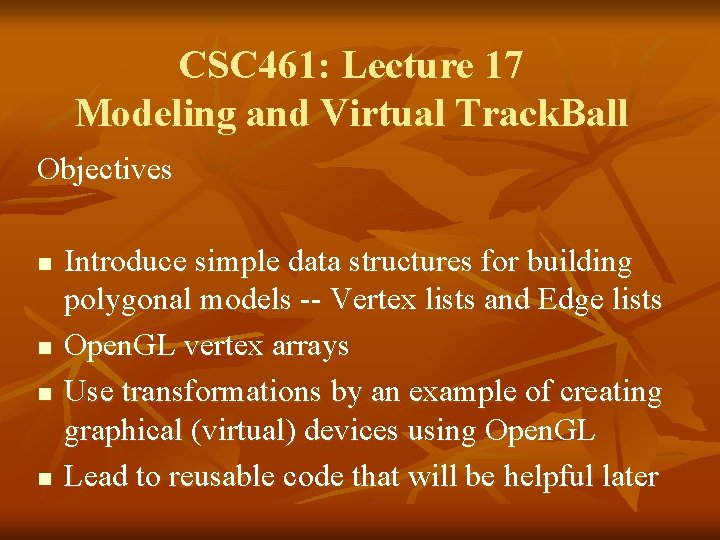CSC 461: Lecture 17 Modeling and Virtual Track. Ball Objectives n n Introduce simple data structures for building polygonal models -- Vertex lists and Edge lists Open. GL vertex arrays Use transformations by an example of creating graphical (virtual) devices using Open. GL Lead to reusable code that will be helpful laterRepresenting a Mesh n e 2 Consider a mesh v 6 e 1 v 1 e 6 n n v 5 e 3 e 9 e 8 v 4 e 11 e 10 v 7 e 4 e 7 v 2 e 12 v 3 e 5 There are 8 nodes and 12 edges n 5 interior polygons n 6 interior (shared) edges Each vertex has a location vi = (xi yi zi)Simple Representation n List all polygons by their geometric locations Leads to Open. GL code such as gl. Begin(GL_POLYGON); gl. Vertex 3 f(x 1, y 1, z 1); gl. Vertex 3 f(x 6, y 6, z 6); gl. Vertex 3 f(x 7, y 7, z 7); gl. End(); Inefficient and unstructured n Consider moving a vertex to a new locations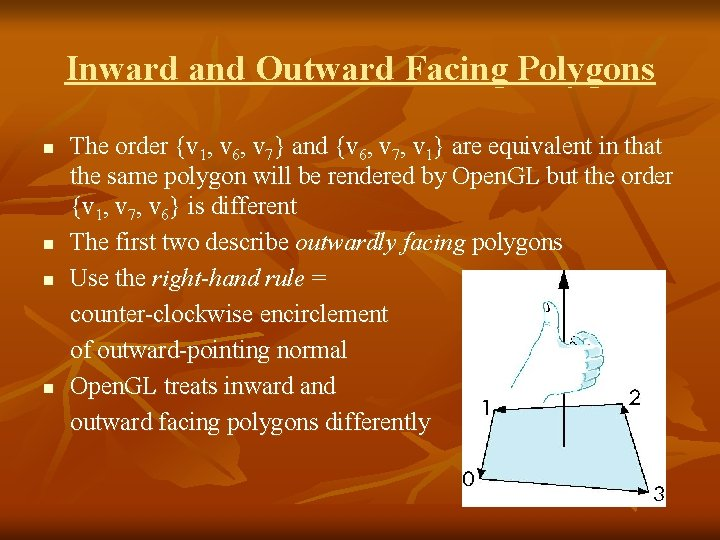Inward and Outward Facing Polygons n n The order {v 1, v 6, v 7} and {v 6, v 7, v 1} are equivalent in that the same polygon will be rendered by Open. GL but the order {v 1, v 7, v 6} is different The first two describe outwardly facing polygons Use the right-hand rule = counter-clockwise encirclement of outward-pointing normal Open. GL treats inward and outward facing polygons differently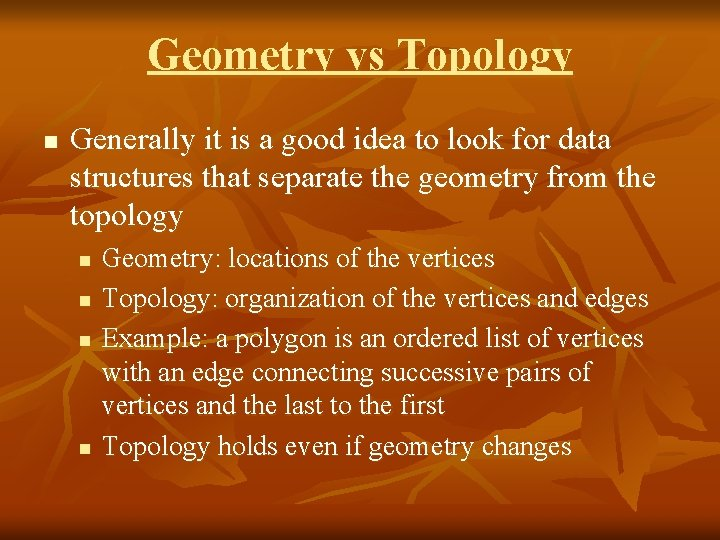Geometry vs Topology n Generally it is a good idea to look for data structures that separate the geometry from the topology n n Geometry: locations of the vertices Topology: organization of the vertices and edges Example: a polygon is an ordered list of vertices with an edge connecting successive pairs of vertices and the last to the first Topology holds even if geometry changes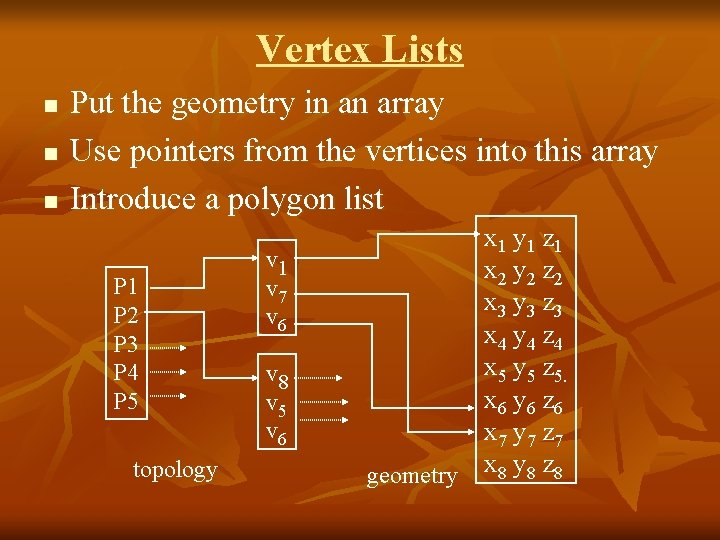Vertex Lists n n n Put the geometry in an array Use pointers from the vertices into this array Introduce a polygon list P 1 P 2 P 3 P 4 P 5 topology v 1 v 7 v 6 v 8 v 5 v 6 x 1 y 1 z 1 x 2 y 2 z 2 x 3 y 3 z 3 x 4 y 4 z 4 x 5 y 5 z 5. x 6 y 6 z 6 x 7 y 7 z 7 geometry x 8 y 8 z 8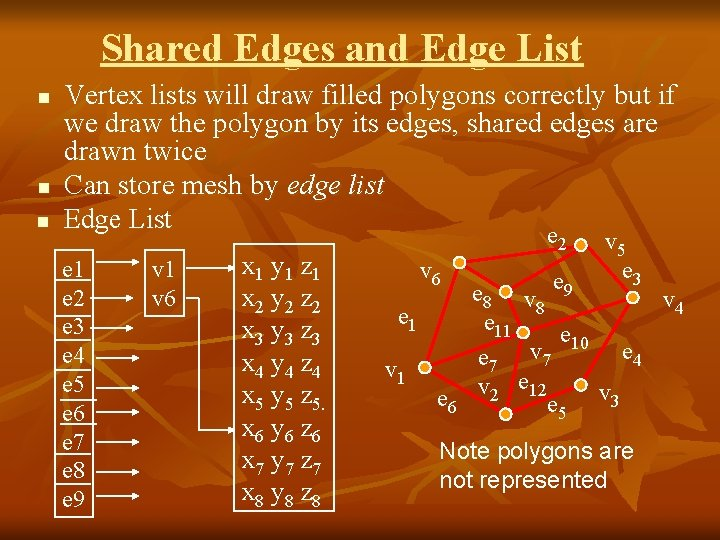Shared Edges and Edge List n n n Vertex lists will draw filled polygons correctly but if we draw the polygon by its edges, shared edges are drawn twice Can store mesh by edge list Edge List e e 1 e 2 e 3 e 4 e 5 e 6 e 7 e 8 e 9 v 1 v 6 x 1 y 1 z 1 x 2 y 2 z 2 x 3 y 3 z 3 x 4 y 4 z 4 x 5 y 5 z 5. x 6 y 6 z 6 x 7 y 7 z 7 x 8 y 8 z 8 2 v 6 e 1 v 1 e 6 v 5 e 3 e 8 v e 9 v 4 8 e 11 e 10 e 4 e 7 v 2 e 12 e 5 v 3 Note polygons are not representedModeling a Cube n n Model a color cube for rotating cube program Define global arrays for vertices and colors GLfloat vertices[] = {{-1. 0, -1. 0}, {-1. 0, 1. 0}, { 1. 0, 1. 0}, {-1. 0, 1. 0}}; GLfloat colors[] = {{0. 0, 0. 0}, {1. 0, 0. 0}, {0. 0, 1. 0}, {1. 0, 1. 0}, {0. 0, 1. 0}}; Drawing a polygon from a list of indices n Draw a quadrilateral from a list of indices into the array vertices and use color corresponding to first index void polygon(int a, int b, int c , int d) { gl. Begin(GL_POLYGON); gl. Color 3 fv(colors[a]); gl. Vertex 3 fv(vertices[b]); gl. Vertex 3 fv(vertices[c]); gl. Vertex 3 fv(vertices[d]); gl. End(); }Draw cube from faces void colorcube( ) { polygon(0, 3, 2, 1); polygon(2, 3, 7, 6); polygon(0, 4, 7, 3); polygon(1, 2, 6, 5); polygon(4, 5, 6, 7); polygon(0, 1, 5, 4); } n n n 5 n n 2 1 Note that vertices are ordered so that 0 we obtain correct outward facing normals Efficiency 6 7 4 3 The weakness of our approach is that we are building the model in the application and must do many function calls to draw the cube Drawing a cube by its faces in the most straight forward way requires n n 6 gl. Begin, 6 gl. End 6 gl. Color 24 gl. Vertex More if we use texture and lightingVertex Arrays n n Open. GL provides a facility called vertex arrays that allow us to store array data in the implementation Six types of arrays supported n n n n Vertices Color indices Normals Texture coordinates Edge flags We will need only colors and vertices Initialization -- Using the same color and vertex data, first we enable gl. Enable. Client. State(GL_COLOR_ARRAY); gl. Enable. Client. State(GL_VERTEX_ARRAY);Using Arrays n Identify location of arrays gl. Vertex. Pointer(3, GL_FLOAT, 0, vertices); data array stored as floats data contiguous 3 d arrays gl. Color. Pointer(3, GL_FLOAT, 0, colors); n Map indices to faces n n Form an array of face indices GLubyte cube. Indices = { 0, 3, 2, 1, 2, 3, 7, 6, 0, 4, 7, 3, 1, 2, 6, 5, 4, 5, 6, 7, 0, 1, 5, 4}; Each successive four indices describe a face of the cubeDrawing the cube n n Draw through gl. Draw. Elements which replaces all gl. Vertex and gl. Color calls in the display callback Method 1: what to draw number of indices for(i=0; i<6; i++) gl. Draw. Elements(GL_POLYGON, 4, GL_UNSIGNED_BYTE, &cube. Indices[4*i]); format of index data n Method 2: start of index data gl. Draw. Elements(GL_QUADS, 24, GL_UNSIGNED_BYTE, cube. Indices); Draws cube with 1 function call!!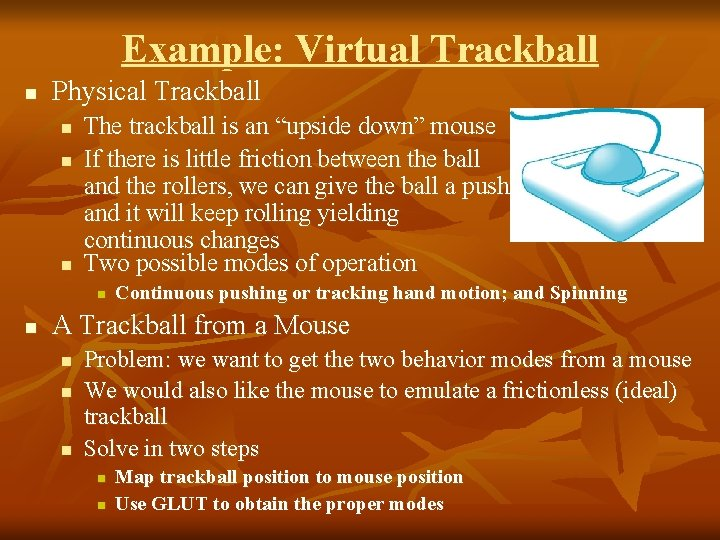Example: Virtual Trackball n Physical Trackball n n n The trackball is an “upside down” mouse If there is little friction between the ball and the rollers, we can give the ball a push and it will keep rolling yielding continuous changes Two possible modes of operation n n Continuous pushing or tracking hand motion; and Spinning A Trackball from a Mouse n n n Problem: we want to get the two behavior modes from a mouse We would also like the mouse to emulate a frictionless (ideal) trackball Solve in two steps n n Map trackball position to mouse position Use GLUT to obtain the proper modes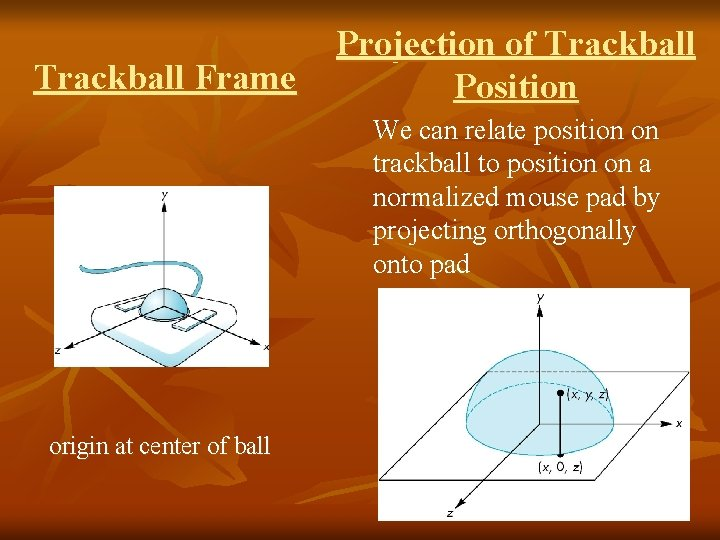Trackball Frame Projection of Trackball Position We can relate position on trackball to position on a normalized mouse pad by projecting orthogonally onto pad origin at center of ball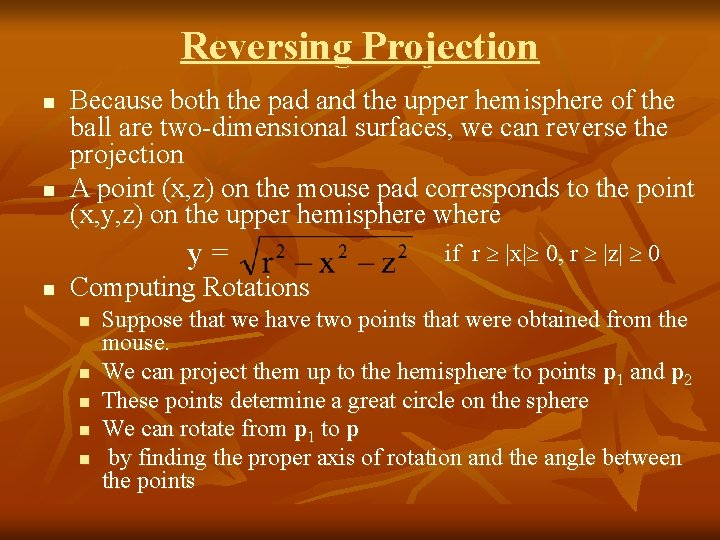Reversing Projection n n Because both the pad and the upper hemisphere of the ball are two-dimensional surfaces, we can reverse the projection A point (x, z) on the mouse pad corresponds to the point (x, y, z) on the upper hemisphere where y= n if r |x| 0, r |z| 0 Computing Rotations n n n Suppose that we have two points that were obtained from the mouse. We can project them up to the hemisphere to points p 1 and p 2 These points determine a great circle on the sphere We can rotate from p 1 to p by finding the proper axis of rotation and the angle between the pointsObtaining the angle n n The axis of rotation is given by the normal to the plane determined by the origin, p 1 , and p 2 The angle between p 1 and p 2 is given by n = p 1 | sin q| = n If we move the mouse slowly or sample its position frequently, then q will be small and we can use the approximation sin q qImplementing with GLUT n n n We will use the idle, motion, and mouse callbacks to implement the virtual trackball Define actions in terms of three booleans tracking. Mouse: if true update trackball position redraw. Continue: if true, idle function posts a redisplay trackball. Move: if true, update rotation matrix In this example, we use the virtual trackball to rotate the color cube we modeled earlier The code for the colorcube function is omitted because it is unchanged from the earlier examples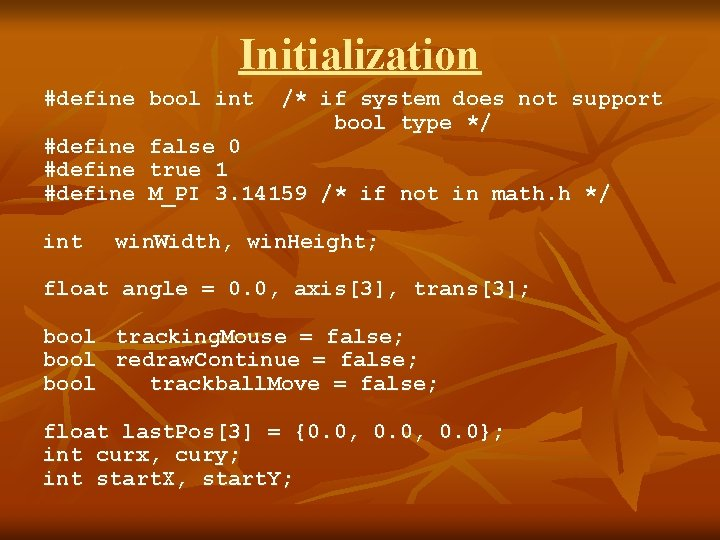Initialization #define bool int /* if system does not support bool type */ #define false 0 #define true 1 #define M_PI 3. 14159 /* if not in math. h */ int win. Width, win. Height; float angle = 0. 0, axis, trans; bool tracking. Mouse = false; bool redraw. Continue = false; bool trackball. Move = false; float last. Pos = {0. 0, 0. 0}; int curx, cury; int start. X, start. Y;The Projection Step voidtrackball_ptov(int x, int y, int width, int height, float v) { float d, a; /* project x, y onto a hemisphere centered within width, height , note z is up here*/ v = (2. 0*x - width) / width; v = (height - 2. 0 F*y) / height; d = sqrt(v*v + v*v); v = cos((M_PI/2. 0) * ((d < 1. 0) ? d : 1. 0)); a = 1. 0 / sqrt(v*v + v*v + v*v); v *= a; v *= a; v *= a; }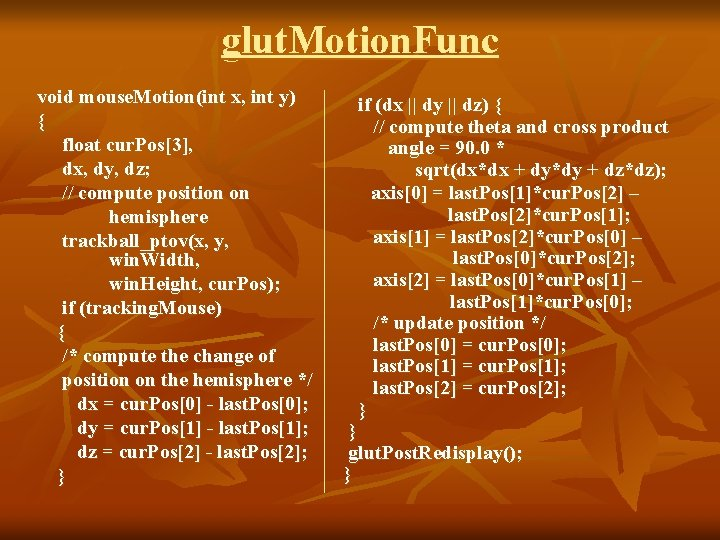glut. Motion. Func void mouse. Motion(int x, int y) { float cur. Pos, dx, dy, dz; // compute position on hemisphere trackball_ptov(x, y, win. Width, win. Height, cur. Pos); if (tracking. Mouse) { /* compute the change of position on the hemisphere */ dx = cur. Pos - last. Pos; dy = cur. Pos - last. Pos; dz = cur. Pos - last. Pos; } if (dx || dy || dz) { // compute theta and cross product angle = 90. 0 * sqrt(dx*dx + dy*dy + dz*dz); axis = last. Pos*cur. Pos – last. Pos*cur. Pos; axis = last. Pos*cur. Pos – last. Pos*cur. Pos; axis = last. Pos*cur. Pos – last. Pos*cur. Pos; /* update position */ last. Pos = cur. Pos; last. Pos = cur. Pos; last. Pos = cur. Pos; } } glut. Post. Redisplay(); }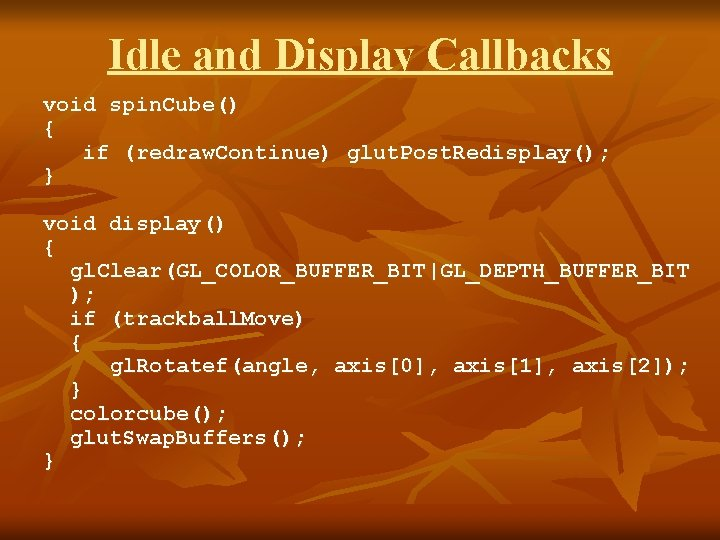Idle and Display Callbacks void spin. Cube() { if (redraw. Continue) glut. Post. Redisplay(); } void display() { gl. Clear(GL_COLOR_BUFFER_BIT|GL_DEPTH_BUFFER_BIT ); if (trackball. Move) { gl. Rotatef(angle, axis, axis, axis); } colorcube(); glut. Swap. Buffers(); }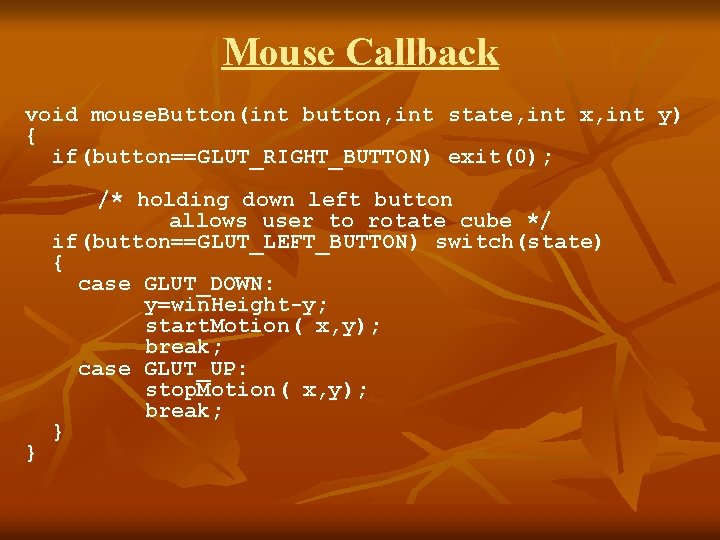Mouse Callback void mouse. Button(int button, int state, int x, int y) { if(button==GLUT_RIGHT_BUTTON) exit(0); } /* holding down left button allows user to rotate cube */ if(button==GLUT_LEFT_BUTTON) switch(state) { case GLUT_DOWN: y=win. Height-y; start. Motion( x, y); break; case GLUT_UP: stop. Motion( x, y); break; }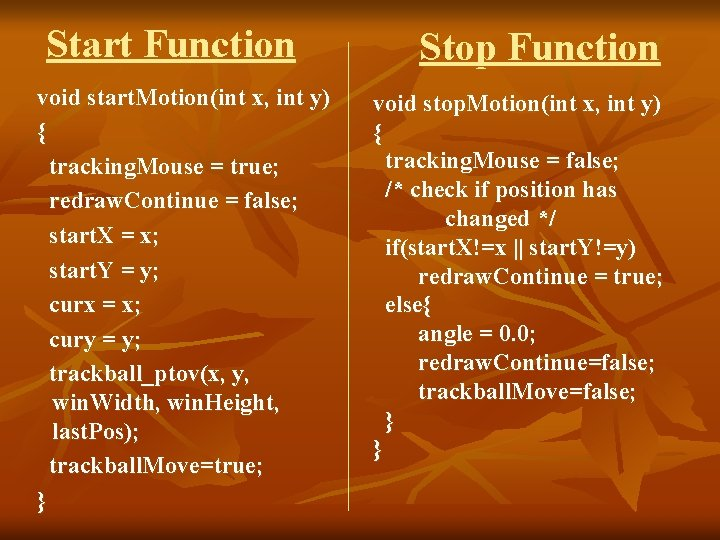Start Function void start. Motion(int x, int y) { tracking. Mouse = true; redraw. Continue = false; start. X = x; start. Y = y; curx = x; cury = y; trackball_ptov(x, y, win. Width, win. Height, last. Pos); trackball. Move=true; } Stop Function void stop. Motion(int x, int y) { tracking. Mouse = false; /* check if position has changed */ if(start. X!=x || start. Y!=y) redraw. Continue = true; else{ angle = 0. 0; redraw. Continue=false; trackball. Move=false; } }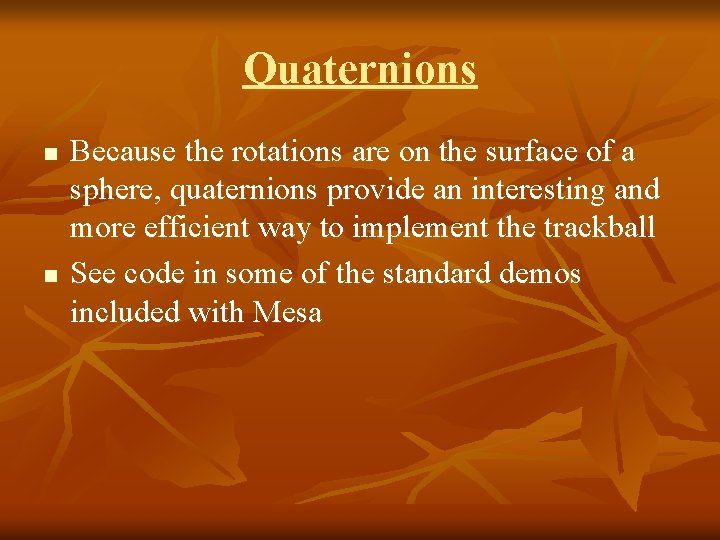Quaternions n n Because the rotations are on the surface of a sphere, quaternions provide an interesting and more efficient way to implement the trackball See code in some of the standard demos included with Mesa